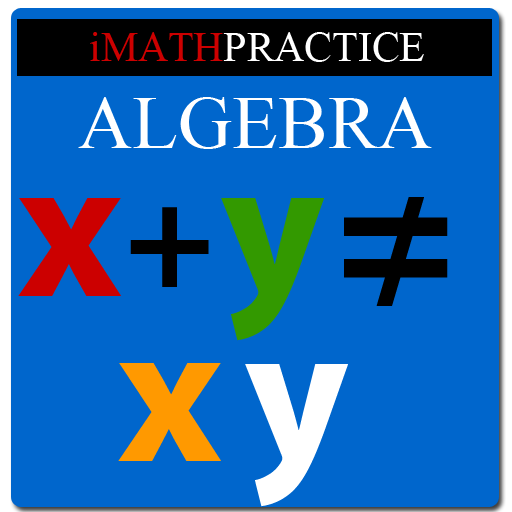# Master Algebra Lite

1.3 for Android
3.7 | 50,000+ Installs | Reviews

ongolearn.com

## Description of Master Algebra Lite

This is for students in High School/College learning algebra. If you are a beginner in algebra you might be thinking X Y=XY, Is not it? But it’s not.
The beauty of algebra is, it deals with variables, expressions & equations. You will come to know various formulas.
For example if you know (a b)^3= a^3 b^3 3a^2b 3ab^2.
You can calculate any number to the powers 2,3,4…in a fraction of seconds.
In the above equation a ,b are variables. So you can calculate (1.034)^3 also using that formula. Just feed a=1& b=.034
IMathPractice Algebra's 3 steps method of teaching has sections like Tutorial, Practice Skills, Practice Test & Algebra Challenge. Under tutorial it teaches you.
Numbers
Types of Number like real number, integer, negative number, complex number
Addition, Subtraction, Multiplication & Division of Real Number
Addition, Subtraction, Multiplication & Division of Negative Number
Addition, Subtraction, Multiplication & Division of Complex Number
Properties of Number
Ratio & Proportion
Integer exponents/real /rational exponents
What is Monomial/binomial/polynomial?
Addition, Subtraction, Multiplication, Division of polynomials
Factoring polynomial
What is variable, expression & equation?
Equation with radical, Absolute value of equation
Rational Expression;
Addition, Subtraction, Multiplication, Division of rational expression
What’s Inequality?
Linear, Polynomial &Rational Inequalities
Absolute value of Inequality
Under Practice Skills, You will be able to practice all the above learned skills with help.
There are answer & steps to get the answer for each question.
Under Practice Test, You will be able to practice all the skills in specified timed environment. There is a timer. You need to finish within that time.
Under Algebra Challenge You will be prepared to compete with others. It contains 50 questions & time allotted is 1 hour.
There are around 210 questions to practice for in lite version.
What's new in version 1.3?
Bug reported in comment section has been removed.
Note:Currently the app is in English only. We are working on translation to other languages. Please don't rate 1 only because it's not in your language.You can send your request to developer.

## Information

• Category:
Education
1.3
• Updated:
2013-11-09
• File size:
523.8KB
• Requirements:
Android 2 or later
• Developer:
ongolearn.com
• ID:
com.eamsol.algebralite
Reviews
••••